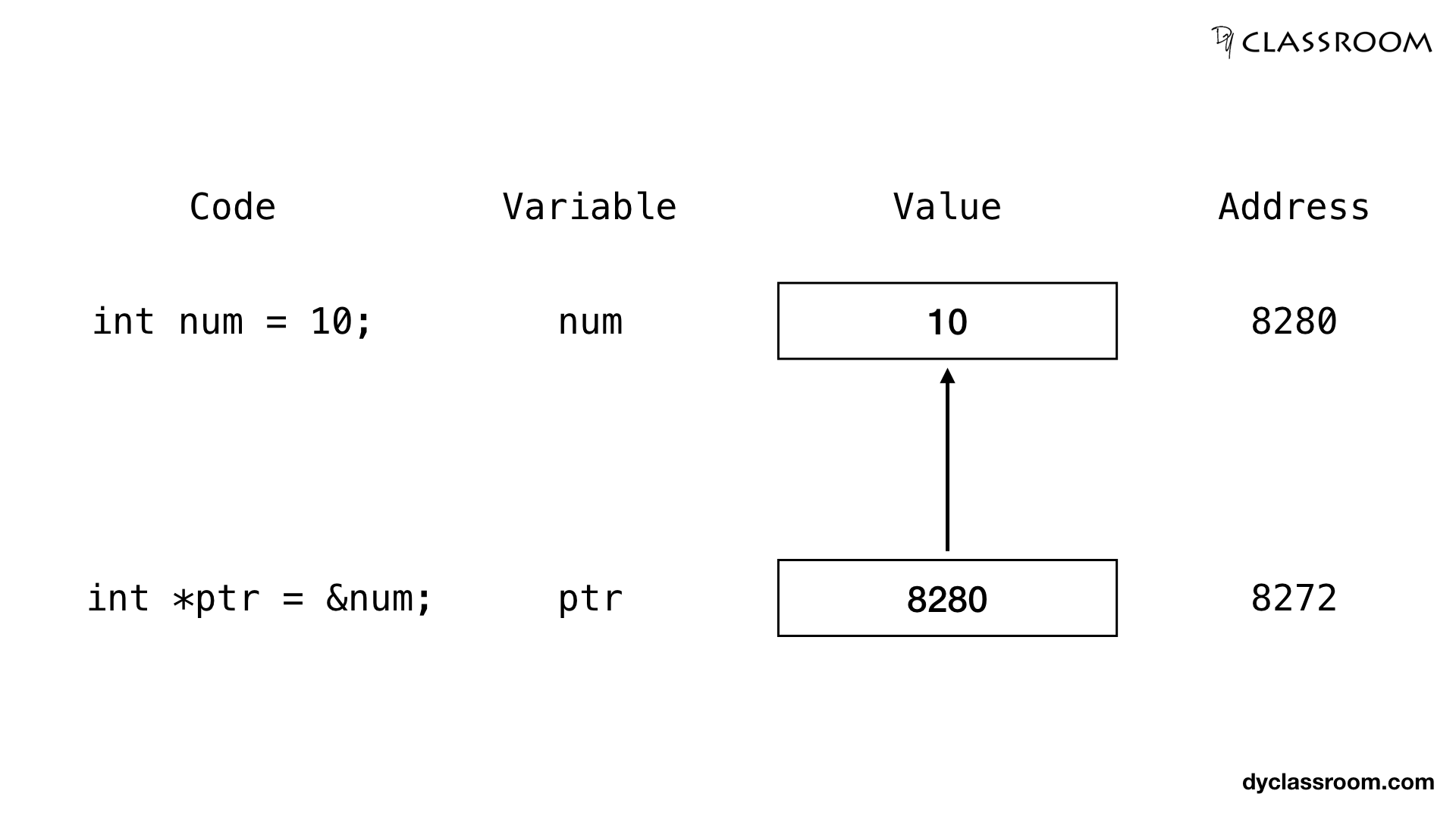# C - Pointers and Variables

C ProgrammingIn this tutorial we will learn to manipulate variables using pointers in C programming language.

We already know from the Pointers tutorial how to create a pointer variable and store address of a variable in it.

Now let us go ahead and create an integer `int` variable and manipulate it via an integer pointer variable `ptr`.

## Creating an integer variable

This is fairly simple. All we have to do is declare a variable using the `int` data type.

``````int num = 10;
``````

Three things will happen for the above line of code.

• A memory location is located to store integer value.
• The value 10 is saved in that memory location.
• We can refer the memory location using the variable name `num`.

## Creating integer pointer variable

Now we will create an integer pointer variable `ptr` that will store the address of the integer variable `num`.

To get the address of a variable we use the address of `&` operator.

``````int *ptr = &num;
``````

We can represent the integer variable `num` and pointer variable `ptr` as follows.So, we can see that variable `num` was allocated memory location 8280 (which will change the next time the program is executed). In that location value 10 was stored.

Similarly, variable `ptr` was allocated memory location 8272 and it holds the value 8280 which is the memory location of variable `num`. So, ptr is pointing at the num variable.

## Accessing value of a variable via pointer

To access the value stored in the variable `num` via the pointer variable `ptr` we have to use the value at the address of `*` operator.

We already have the address of variable `num` stored in variable `ptr`. So, using `*ptr` will give use the value stored at the address i.e., value stored in num variable.

``````//value of num via ptr
printf("num value via ptr: %d\n", *ptr);
``````

## Updating the value of a variable via pointer

To update the value of a variable via pointer we have to first get the address of the variable and then set the new value in that memory address.

We get the address via address of `&` operator and then we set the value using the value at the address of `*` operator.

``````// updating the value of num via ptr
*ptr = 20;
``````

Complete code:

``````#include <stdio.h>

int main(void) {

// num variable
int num = 10;

// ptr pointer variable
int *ptr = NULL;

// assigning the address of num to ptr
ptr = &num;

// printing the value of num - Output: 10
printf("num: %d\n", num);
printf("num via ptr: %d\n", *ptr);

// updating the value of num via ptr
printf("Updating value of num via ptr...\n");
*ptr = 20;

// printing the new value of num - Output: 20
printf("num: %d\n", num);
printf("num via ptr: %d\n", *ptr);

return 0;
}
``````

Output:

``````num: 10
num via ptr: 10
Updating value of num via ptr...
num: 20
num via ptr: 20
``````